# Chapter 3 Analysis

“First lesson, stick them with the pointy end.”

— Jon Snow

Previous chapters focused on introducing Spark with R, they got you up to speed and encouraged you to try basic data analysis workflows. However, they have not properly introduced what such data analysis means, especially while running in Spark. They presented the tools you need throughout this book, to help you spend more time learning and less time troubleshooting.

This chapter will introduce tools and concepts to perform data analysis in Spark from R; which, spoiler alert, these are the same tools you use when using plain R! This is not accidental coincidence; but rather, we want data scientist to live in a world where technology is hidden from them, where you can use the R packages you know and love, and where they simply happen to just work in Spark! Now, we are not quite there yet, but we are also not that far. Therefore, in this chapter you will learn widely used R packages and practices to pereform data analysis like: dplyr, ggplot2, formulas, rmarkdown and so on – which also happen to work in Spark!

The next chapter, Modeling, will focus on creating statistical models to predict, estimate and describe datasets; but first, let’s get started with analysis!

## 3.1 Overview

In a data analysis project, the main goal is to understand what the data is trying to “tell us”, hoping that it provides an answer to a specific question. Most data analysis projects follow a set of steps outlined in Figure ??.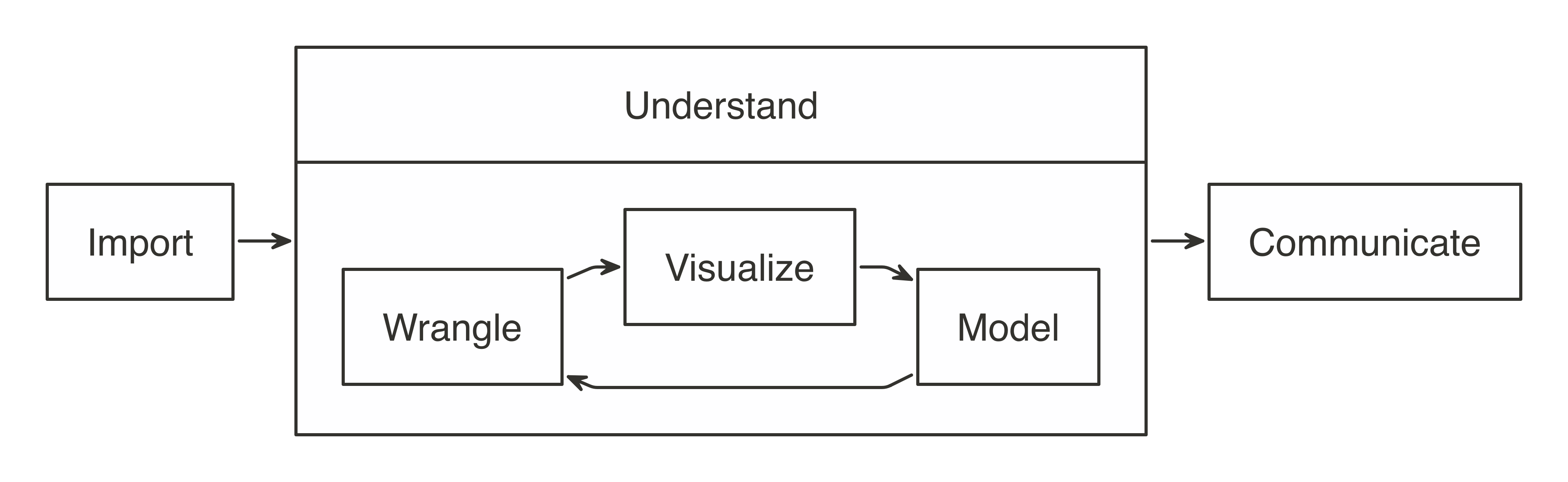General steps of a data analysis||analysis-steps

As the diagram illustrates, we first import data into our analysis stem, then wrangled by trying different data transformations, such as aggregations, and then visualized to help us perceive relationships and trends. In order to get deeper insight, one or multiple statistical models can be fitted against sample data. This will help in finding out if the patterns hold true when new data is applied to them. And lastly, the results are communicated publicly or privately to colleagues and stakeholders.

When working with not-large-scale datasets, as in datasets that fit in memory; we can perform all those steps from R, without using Spark. However, when data does not fit in memory or computation is simply too-slow, we can slightly modify this approach by incorporating Spark, but how?

For data analysis, the ideal approach is to let Spark do what its good at. Spark is a parallel computation engine that works at a large-scale and provides a SQL engine and modeling libraries. These can be used to perform most of the same operations R performs. Such operations include data selection, transformation, and modeling. Additionally, Spark includes Graph analysis and Streaming libraries and many other; for now, we will skip those non-rectangular datasets and present them in later chapters.

Data import, wrangling, and modeling can be performed inside Spark. Visualization can also partly be done by Spark, we will cover that later in this chapter. The idea is to use R to tell Spark what data operations to run, and then only bring the results into R. As illustrated in Figure ??, the ideal method pushes compute to the Spark cluster, and then collects results into R.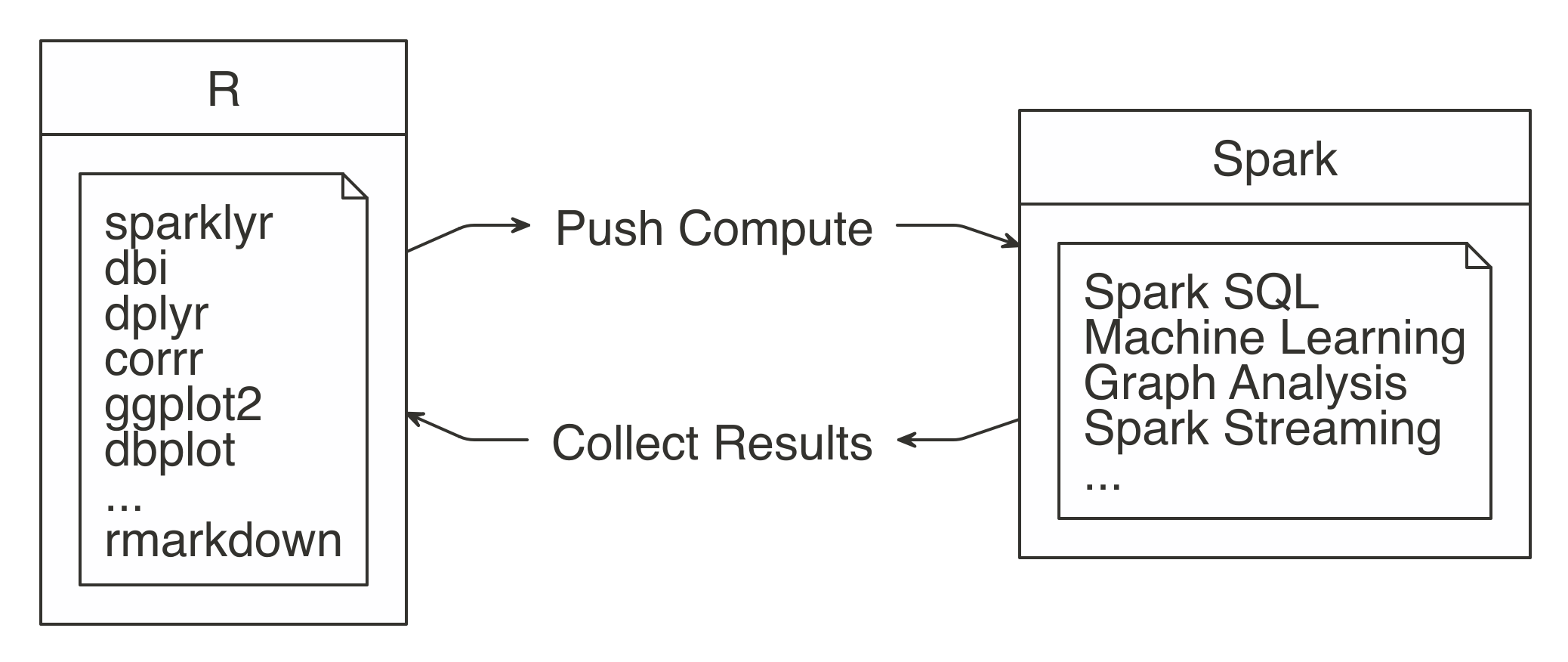Spark computes while R collects results||analysis-approach

The sparklyr package aids in using the “push compute, collect results” principle. Most of its functions are mainly wrappers on top of Spark API calls. This allows us to take advantage of Spark’s analysis components, instead of R’s. For example, when you need to fit a linear regression model; instead of using R’s familiar lm() function, you would use Spark’s ml_linear_regression(). This R function then calls Spark to create this model, this specific example is illustrated in Figure ??.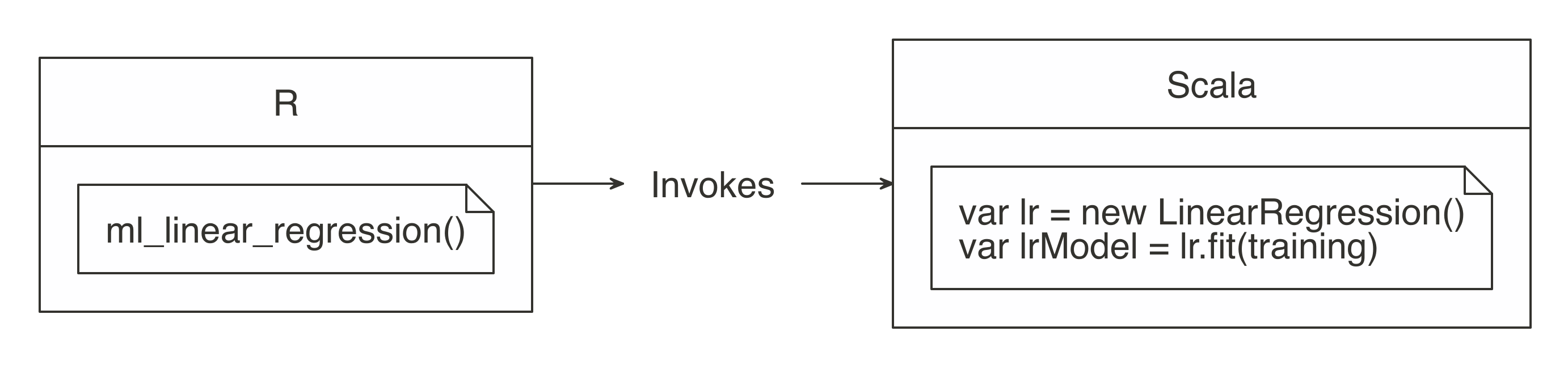R functions call Spark functionality||analysis-scala

For more common data manipulation tasks, sparklyr provides a back-end for dplyr. This means that already familiar dplyr verbs can be used in R, and then sparklyr and dplyr will translate those actions into Spark SQL statements, which are almost certainly much more compact and easier to read than SQL statements. So, if you are already familiar with R and dplyr, there is nothing new to learn! This might feel a bit anticlimactic, it is indeed, but it’s also great since you can focus that energy on learning other skills required to do large-scale computing.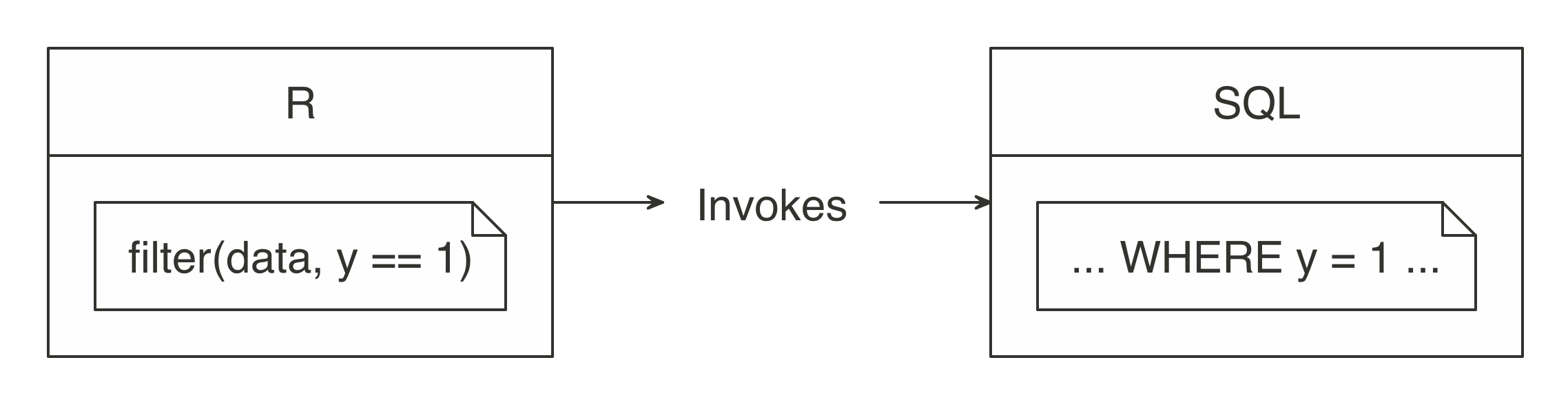dplyr writes SQL||unnamed-chunk-23

In order to practice as you learn, the rest of this chapter’s code will use a single exercise that runs in the local Spark master. This way, the code can be replicated in your personal computer. Please, make sure to already have sparklyr working, this should already be the case if you completed the Getting Started chapter.

This chapter will make use of packages that you might not have installed; so first, make sure the following packages are installed by running:

install.packages("ggplot2")
install.packages("corrr")
install.packages("dbplot")
install.packages("rmarkdown")

First, load the sparklyr and dplyr packages, and open a new local connection.

library(sparklyr)
library(dplyr)

sc <- spark_connect(master = "local", version = "2.3")

The environment is ready to be used, so our next task is to import data that we can later analyze.

## 3.2 Import

Importing data is to be approached differently when using Spark with R. Usually, importing means that R will read files and load them into memory; when using Spark, the data is imported into Spark, not R. Notice how in Figure ?? the data source is connected to Spark instead of being connected to R.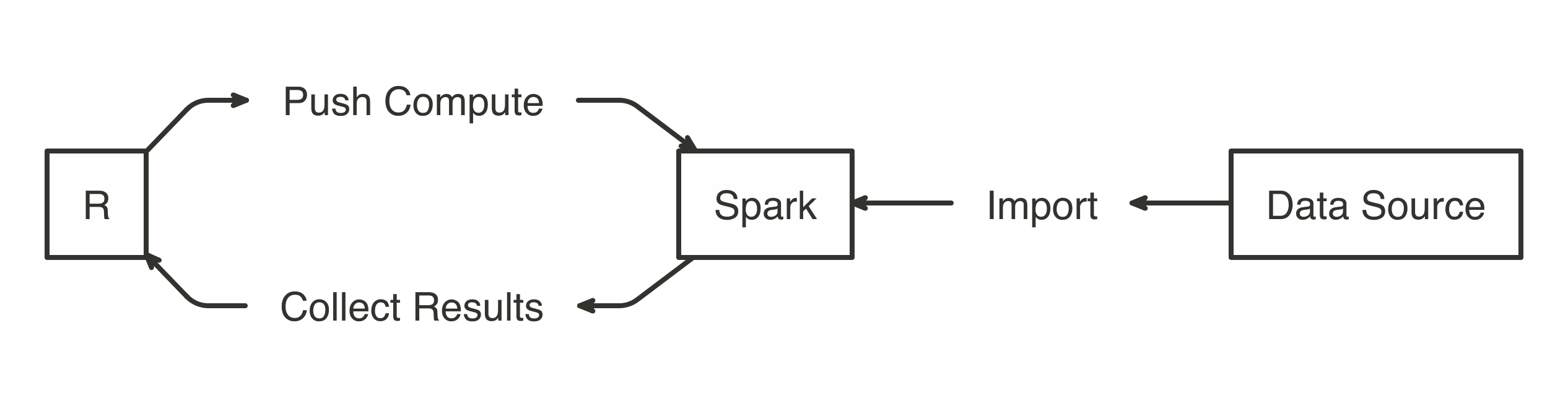Import Data to Spark not R||analysis-access

Note: When you doing analysis over large-scale datasets, the vast majority of the necessary data will be already available in your Spark cluster (which is usually made available to users via Hive tables, or by accessing the file system directly), the Data chapter will cover this extensively.

Rather than importing all data into Spark, you can also request Spark to access the data source without importing it – this is a decision you should make based on speed and performance. Importing all of the data into the Spark session will incur a up-front cost, once; since Spark needs to wait for the data to be loaded before analyzing it. If the data is not imported, you usually incur a cost with every Spark operation since Spark needs to retrieve a subset from the cluster’s storage, which is usually disk drives that happen to be much slower than reading from Spark’s memory. More will be covered in the Tuning chapter.

Let’s prime the session with some data by importing mtcars into Spark using copy_to(); you can also import data from distributed files in many different file formats, which you’ll learn in the Data chapter.

cars <- copy_to(sc, mtcars)

Note: In an enterprise setting, copy_to() should only be used to transfer small tables from R, large data transfers should be performed with specialized data transfer tools.

The data is now accessible to Spark and transformations can now be applied with ease; the next section will cover how to wrangle data by running transformations inside Spark, using dplyr.

## 3.3 Wrangle

Data wrangling uses transformations to understand the data, it is often referred to as the process of transforming data from one “raw” data form into another format with the intent of making it more appropriate for data analysis.

Malformed or missing values and columns with multiple attributes are common data problems you might need to fix, since they prevent you from understanding your dataset. For example, a “name” field contains the last and first name of a customer. There are two attributes (first and last name) in a single column. In order to be usable, we need to transform the “name” field, by changing it into “first_name” and “last_name” fields.

After the data is cleaned, you still need to understand the basics about its content. Other transformations, such as aggregations, can help with this task. For example, the result of requesting the average balance of all customers will return a single row and column. The value will be the average of all customers. That information will give us context when we see individual, or grouped, customer balances.

The main goal is to write the data transformations using R syntax as much as possible. This saves us from the cognitive cost of having to switch between multiple computer technologies to accomplish a single task. In this case, it is better to take advantage of dplyr, instead of writing Spark SQL statements for data exploration.

In the R environment, cars can be treated as if it is a local data frame, so dplyr verbs can be used. For instance, we can find out the mean of all columns as with summarise_all():

summarize_all(cars, mean)
# Source: spark<?> [?? x 11]
mpg   cyl  disp    hp  drat    wt  qsec    vs    am  gear  carb
<dbl> <dbl> <dbl> <dbl> <dbl> <dbl> <dbl> <dbl> <dbl> <dbl> <dbl>
1  20.1  6.19  231.  147.  3.60  3.22  17.8 0.438 0.406  3.69  2.81

While this code is exactly the same as the code you would run when using dplyr without Spark, a lot is happening under the hood! The data is NOT being imported into R; instead,dplyr converts this task into SQL statements that are then sent to Spark. The show_query() command makes it possible to peer into the SQL statement that sparklyr and dplyr created and sent to Spark. We can also use this time to introduce the pipe (%>%) operator, a custom operator from the magrittr package that takes pipes a computation into the first argument of the next function, making your data analysis much easier to read.

summarize_all(cars, mean) %>%
show_query()
<SQL>
SELECT AVG(mpg) AS mpg, AVG(cyl) AS cyl, AVG(disp) AS disp,
AVG(hp) AS hp, AVG(drat) AS drat, AVG(wt) AS wt,
AVG(qsec) AS qsec, AVG(vs) AS vs, AVG(am) AS am,
AVG(gear) AS gear, AVG(carb) AS carb
FROM mtcars

As it is evident, dplyr is much more concise than SQL; but rest assured, you will not have to see nor understand SQL when using dplyr. Your focus can remain on obtaining insights from the data, as opposed to figuring out how to express a given set of transformation in SQL. Here is another example that groups the cars dataset by “transmission” type.

cars %>%
mutate(transmission = ifelse(am == 0, "automatic", "manual")) %>%
group_by(transmission) %>%
summarise_all(mean)
# Source: spark<?> [?? x 12]
transmission   mpg   cyl  disp    hp  drat    wt  qsec    vs    am  gear  carb
<chr>        <dbl> <dbl> <dbl> <dbl> <dbl> <dbl> <dbl> <dbl> <dbl> <dbl> <dbl>
1 automatic     17.1  6.95  290.  160.  3.29  3.77  18.2 0.368     0  3.21  2.74
2 manmual       24.4  5.08  144.  127.  4.05  2.41  17.4 0.538     1  4.38  2.92

Most of the data transformation made available by dplyr to work with local data frames are also available to use with a Spark connection. This means that you can focus on learning dplyr first, and then reuse that skill when working with Spark. The Data Transformation chapter from the “R for Data Science” (Wickham and Grolemund 2016) book is a great resource to learn in-depth dplyr. If proficiency with dplyr is not an issue for you, then please take some time to experiment with different dplyr functions against the cars table.

Sometimes we may need to perform an operation not yet available through dplyr and sparklyr. Instead of downloading the data into R, there is usually a Hive function within Spark to accomplish what we need. The next section will cover this scenario.

### 3.3.1 Built-in Functions

Spark SQL is based on Hive’s SQL conventions and functions and it is possible to call all these functions using dplyr as well. This means that we can use any Spark SQL functions to accomplish operations that may not be available via dplyr. The functions can be accessed by calling them as if they were R functions. Instead of failing, dplyr passes functions it does not recognize “as-is” to the query engine. This gives us a lot of flexibility on the function we can use!

For instance, the percentile function returns the exact percentile of a column in a group. The function expects a column name, and either a single percentile value, or an array of multiple percentile values. We can use this Spark SQL function from dplyr as follows:

summarise(cars, mpg_percentile = percentile(mpg, 0.25))
# Source: spark<?> [?? x 1]
mpg_percentile
<dbl>
1           15.4

There is no percentile() function in R, so dplyr passes the that portion of the code, “as-is”, to the resulting SQL query.

summarise(cars, mpg_percentile = percentile(mpg, 0.25)) %>%
show_query()
<SQL>
SELECT percentile(mpg, 0.25) AS mpg_percentile
FROM mtcars_remote

To pass multiple values to percentile, we can call another Hive function called array. In this case, array would work similarly to R’s list() function. We can pass multiple values separated by commas. The output from Spark is an array variable, which is imported into R as a list variable column.

summarise(cars, mpg_percentile = percentile(mpg, array(0.25, 0.5, 0.75)))
# Source: spark<?> [?? x 1]
mpg_percentile
<list>
1 <list >   

The explode function can be used to separate the Spark’s array value results into their own record. To do this, use explode within a mutate() command, and pass the variable containing the results of the percentile operation.

summarise(cars, mpg_percentile = percentile(mpg, array(0.25, 0.5, 0.75))) %>%
mutate(mpg_percentile = explode(mpg_percentile))
# Source: spark<?> [?? x 1]
mpg_percentile
<dbl>
1           15.4
2           19.2
3           22.8

We have included a comprehensive list of all the Hive functions in the Appendix under Hive functions, make sure you glance over them to get a sense of the wide range of operations you can accomplish with them.

### 3.3.2 Correlations

A very common exploration technique is to calculate and visualize correlations, which we often calculate to find out what kind of statistical relationship exists between paired sets of variables. Spark provides functions to calculate correlations across the entire data set and returns the results to R as a data frame object.

ml_corr(cars)
# A tibble: 11 x 11
mpg    cyl   disp     hp    drat     wt    qsec
<dbl>  <dbl>  <dbl>  <dbl>   <dbl>  <dbl>   <dbl>
1  1     -0.852 -0.848 -0.776  0.681  -0.868  0.419
2 -0.852  1      0.902  0.832 -0.700   0.782 -0.591
3 -0.848  0.902  1      0.791 -0.710   0.888 -0.434
4 -0.776  0.832  0.791  1     -0.449   0.659 -0.708
5  0.681 -0.700 -0.710 -0.449  1      -0.712  0.0912
6 -0.868  0.782  0.888  0.659 -0.712   1     -0.175
7  0.419 -0.591 -0.434 -0.708  0.0912 -0.175  1
8  0.664 -0.811 -0.710 -0.723  0.440  -0.555  0.745
9  0.600 -0.523 -0.591 -0.243  0.713  -0.692 -0.230
10  0.480 -0.493 -0.556 -0.126  0.700  -0.583 -0.213
11 -0.551  0.527  0.395  0.750 -0.0908  0.428 -0.656
# ... with 4 more variables: vs <dbl>, am <dbl>,
#   gear <dbl>, carb <dbl>

The corrr R package specializes in correlations. It contains friendly functions to prepare and visualize the results. Included inside the package is a back-end for Spark, so when a Spark object is used in corrr the actual computation also happens in Spark. In the background, the correlate() function runs sparklyr::ml_corr(), so there is no need to collect any data into R prior running the command.

library(corrr)
correlate(cars, use = "pairwise.complete.obs", method = "pearson") 
# A tibble: 11 x 12
rowname     mpg     cyl    disp      hp     drat      wt
<chr>     <dbl>   <dbl>   <dbl>   <dbl>    <dbl>   <dbl>
1 mpg      NA      -0.852  -0.848  -0.776   0.681   -0.868
2 cyl      -0.852  NA       0.902   0.832  -0.700    0.782
3 disp     -0.848   0.902  NA       0.791  -0.710    0.888
4 hp       -0.776   0.832   0.791  NA      -0.449    0.659
5 drat      0.681  -0.700  -0.710  -0.449  NA       -0.712
6 wt       -0.868   0.782   0.888   0.659  -0.712   NA
7 qsec      0.419  -0.591  -0.434  -0.708   0.0912  -0.175
8 vs        0.664  -0.811  -0.710  -0.723   0.440   -0.555
9 am        0.600  -0.523  -0.591  -0.243   0.713   -0.692
10 gear      0.480  -0.493  -0.556  -0.126   0.700   -0.583
11 carb     -0.551   0.527   0.395   0.750  -0.0908   0.428
# ... with 5 more variables: qsec <dbl>, vs <dbl>,
#   am <dbl>, gear <dbl>, carb <dbl>

We can pipe the results to other corrr functions. For example, the shave() functions turns all of the duplicated results into NA’s. Again, while this feels like standard R code using existing R packages, Spark is being used under the hood to perform the correlation!

Additionally, as shown in Figure ??, the results can be easily visualized using the rplot() function.

correlate(cars, use = "pairwise.complete.obs", method = "pearson") %>%
shave() %>%
rplot()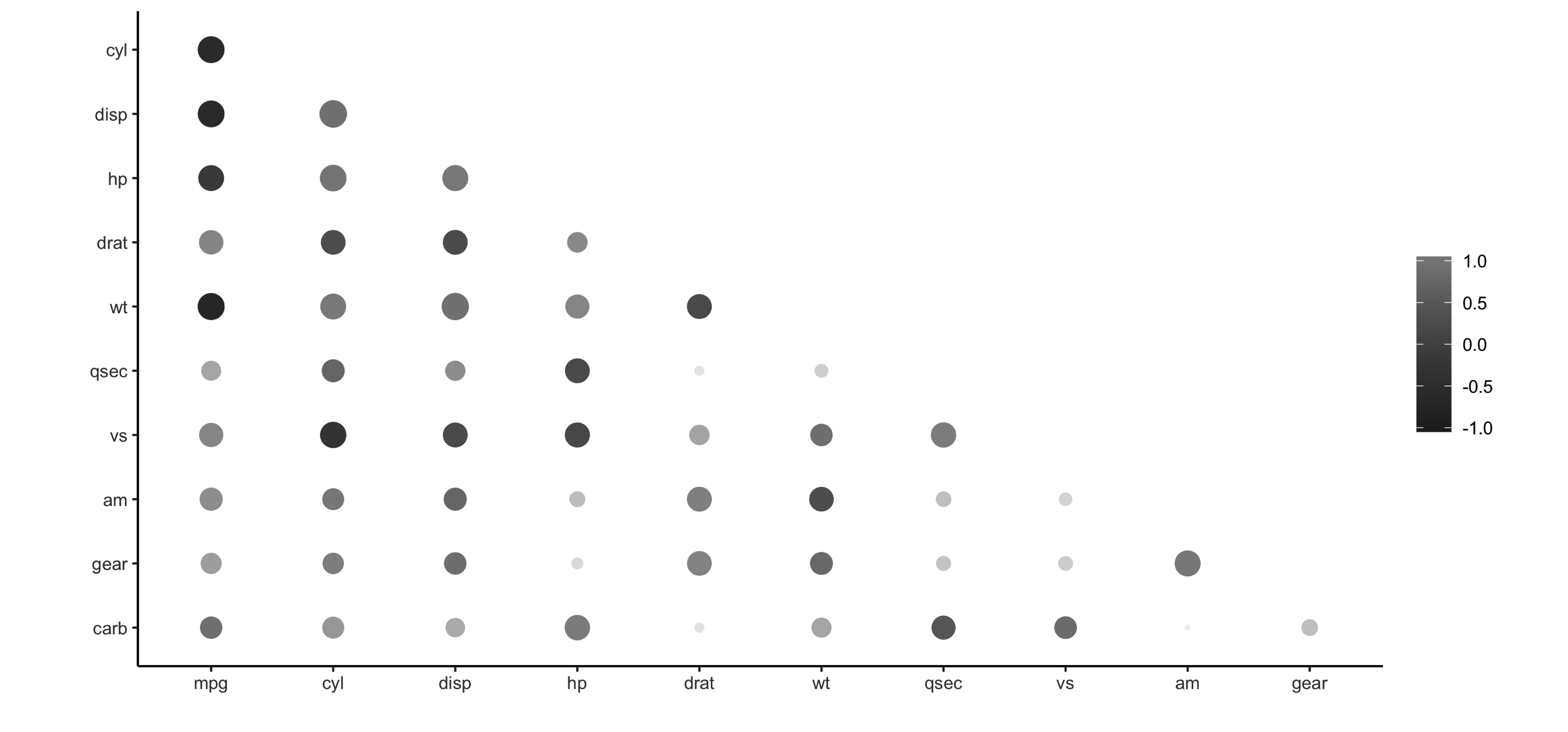Using rplot() to visualize correlations||analysis-corrr-rplot

It is much easier to see which relationships are positive or negative. Positive relationships are in grey, and negative relationships are black. The size of the circle indicates how significant their relationship is. The power of visualizing data is in how much easier it makes it for us to understand results. The next section will expand on this step of the process.

## 3.4 Visualize

Visualizations are a vital tool to help us find patterns in the data. It is easier for us to identify outliers in a data set of 1,000 observations when plotted in a graph, as opposed to reading them from a list.

R is great at data visualizations. Its capabilities for creating plots is extended by the many R packages that focus on this analysis step. Unfortunately, the vast majority of R functions that create plots depend on the data already being in local memory within R, so they fail when using a remote table inside Spark.

It is possible to create visualizations in R from data source from Spark. To understand how to do this, let’s first break down how computer programs build plots: It takes the raw data and performs some sort of transformation. The transformed data is then mapped to a set of coordinates. Finally, the mapped values are drawn in a plot. Figure ?? summarizes each of the steps.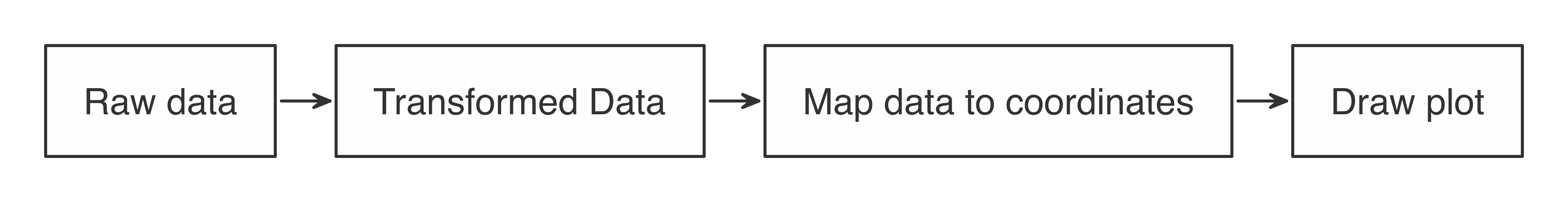Stages of an R plot||analysis-plot

In essence, the approach for visualizing is the same as in wrangling, push the computation to Spark, and then collect the results in R for plotting. As illustrated in Figure ??, the heavy lifting of preparing the data, such as in aggregating the data by groups or bins, can be done inside Spark, and then collect the much smaller data set into R. Inside R, the plot becomes a more basic operation. For example, to plot a histogram, the bins are calculated in Spark, and then in R, use a simple column plot, as opposed to a histogram plot, because there is no need for R to re-calculate the bins.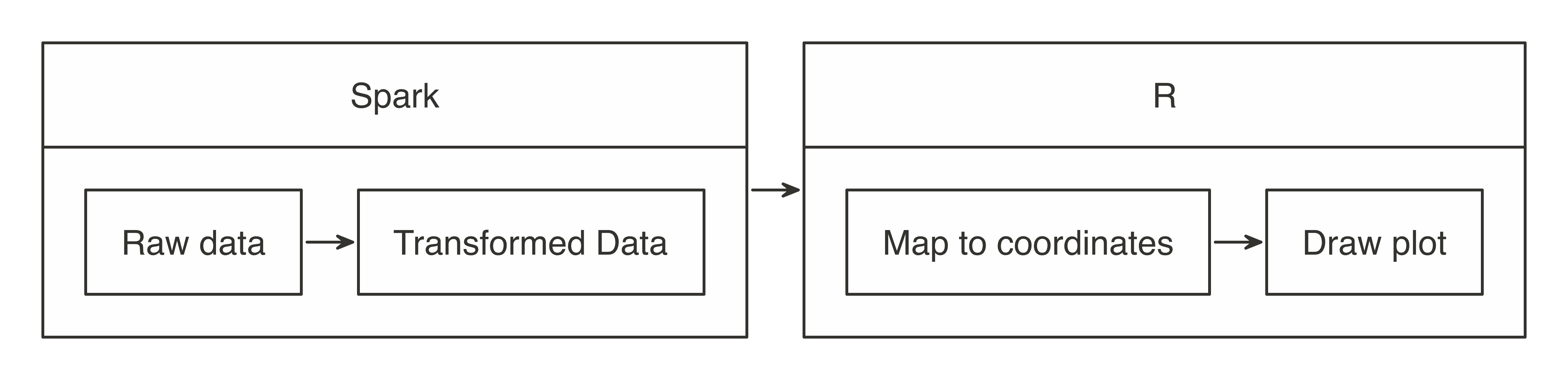Plotting with Spark and R||analysis-spark-plot

Using this conceptual model, let’s apply this when using ggplot2.

### 3.4.1 Using ggplot2

To create a bar plot using ggplot2, we simply call a function:

library(ggplot2)
ggplot(aes(as.factor(cyl), mpg), data = mtcars) + geom_col()FIGURE 3.1: Plotting inside R

In this case, the mtcars raw data was automatically transformed into three discrete aggregated numbers, then each result was mapped into an x and y plane, and then the plot was drawn. As R users, all of the stages of building the plot are conveniently abstracted for us.

In Spark, there are a couple of key steps when codifying the “push compute, collect results” approach. First, ensure that the transformation operations happen inside Spark. In the example below, group_by() and summarise() will run as inside Spark. The second is to bring the results back into R after the data has been transformed. Make sure to transform and then collect, in that order; if collect() is run first, then R will try to ingest the entire data set from Spark. Depending on the size of the data, collecting all of the data will slow down or may even bring down your system.

car_group <- cars %>%
group_by(cyl) %>%
summarise(mpg = sum(mpg, na.rm = TRUE)) %>%
collect() %>%
print()
# A tibble: 3 x 2
cyl   mpg
<dbl> <dbl>
1     6  138.
2     4  293.
3     8  211.

In this example, now that the data has been pre-aggregated and collected into R, only three records are passed to the plotting function. Figure ?? shows the resulting plot.

ggplot(aes(as.factor(cyl), mpg), data = car_group) +
geom_col(fill = "#999999") + coord_flip()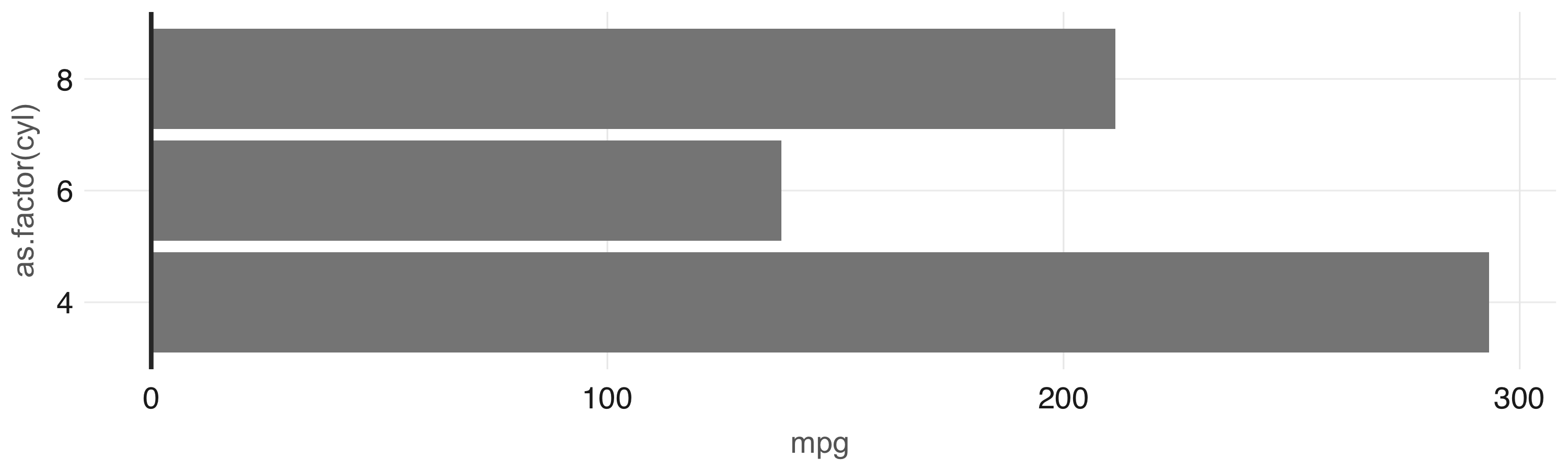Plot with aggregation in Spark||analysis-viz1

Any other ggplot2 visualization can be made to work using this approach; however, is beyond the scope of the book to teach this. Instead, we recommend you use the “R graphics cookbook: practical recipes for visualizing data” (Chang 2012) to learn additional visualization techniques applicable to Spark. Now, to ease this transformation step before visualizing, the dbplot package provides a few read-to-use visualizations that automate aggregation in Spark.

### 3.4.2 Using dbplot

The dbplot package provides helper functions for plotting with remote data. The R code dbplot uses to transform the data is written so that it can be translated into Spark. It then uses those results to create a graph using the ggplot2 package where data transformation and plotting are both triggered by a single function.

The dbplot_histogram() function makes Spark calculate the bins and the count per bin and outputs a ggplot object which can be further refined by adding more steps to the plot object. dbplot_histogram() also accepts a binwidth argument to control the range used to compute the bins, the resulting plot is in Figure ??.

library(dbplot)

cars %>%
dbplot_histogram(mpg, binwidth = 3) +
labs(title = "MPG Distribution",
subtitle = "Histogram over miles per gallon")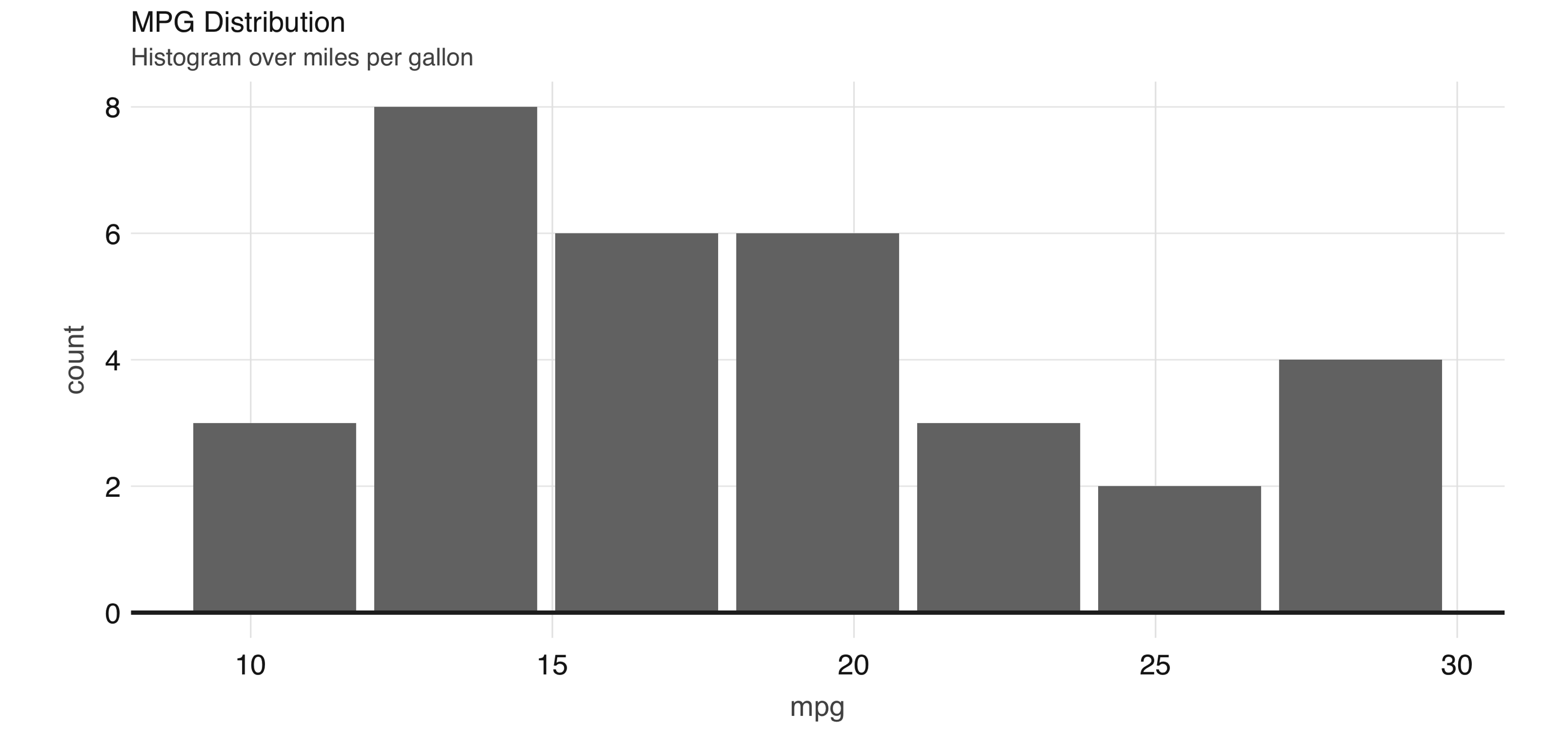Histogram created by dbplot||analysis-visualizations-histogram

Histograms provide a great way to analyze a single variable. To analyze two variables, a scatter or raster plot is commonly used.

Scatter plots are used to compare the relationship between two continuous variables. For example, a scatter plot will display the relationship between the weight of a car and its gas consumption. The plot will show that the higher the weight, the higher the gas consumption because the dots clump together into almost a line that goes from the top left towards the bottom right. See Figure ?? for an example of the plot.

ggplot(aes(mpg, wt), data = mtcars) +
geom_point()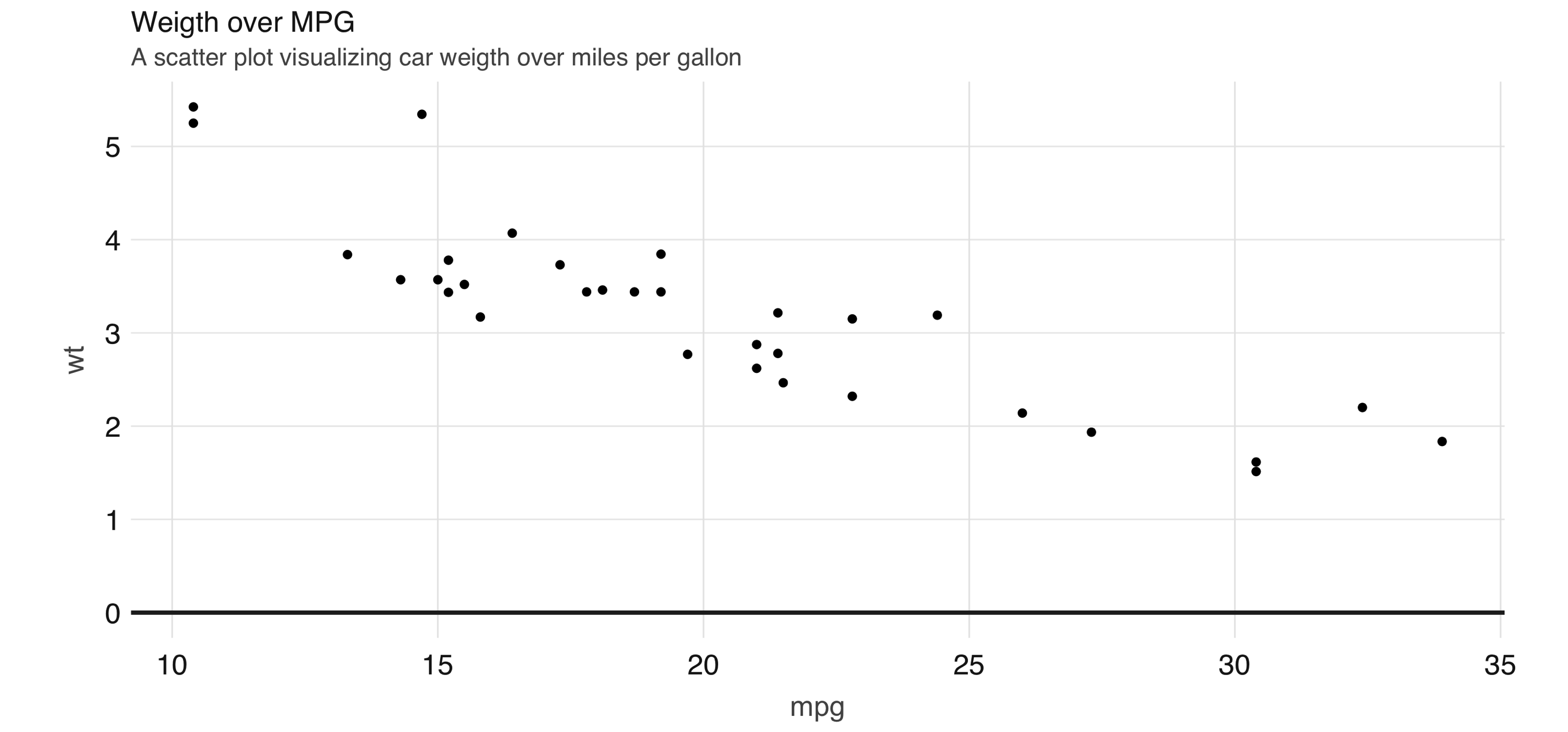Scatter plot example in Spark||analysis-point

However, for scatter plots, no amount of “pushing the computation” to Spark will help with this problem because the data has to be plotted in individual dots.

The best alternative is to find a plot type that represents the x/y relationship and concentration in a way that it is easy to perceive and to “physically” plot. The raster plot may be the best answer. It returns a grid of x/y positions and the results of a given aggregation usually represented by the color of the square.

You can use dbplot_raster() to create a scatter-like plot in Spark, while only retrieving a small subset of the remote dataset:

dbplot_raster(cars, mpg, wt, resolution = 16)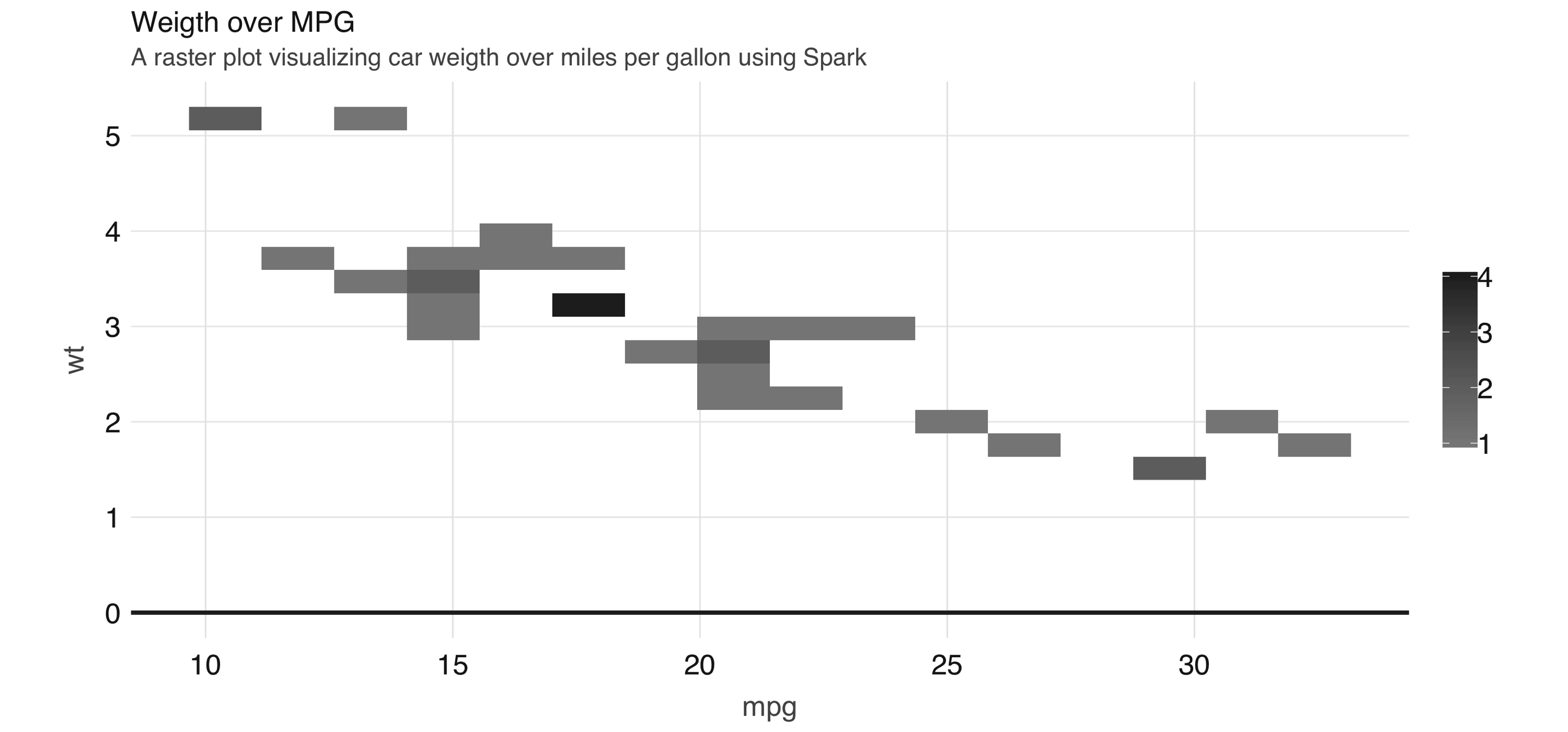A raster plot using Spark||analysis-visualizations-raster

As shown in Figure ??, the plot returns a grid no bigger than 5x5. This limits the number of records that need to be collected into R to 25.

Tip: You can also use dbplot to retrieve the raw data and visualize by other means; to retrieve the aggregates but not the plots use: db_compute_bins(), db_compute_count(), db_compute_raster() and db_compute_boxplot().

While visualizations are indispensable, you can complement data analysis using statistical models to gain even deeper insights into our data. The next section will present how we can prepare data for modeling with Spark.

## 3.5 Model

The next two chapters will focus entirely on modeling, so rather than introducing modeling with too much detail in this chapter, we want to present how to interact with models while doing data analysis.

First, an analysis project goes through as many transformations and models to find the answer. That’s why the first data analysis diagram we introduced in Figure ??, illustrates a cycle between: visualizing, wrangling and modeling – we know you don’t end with modeling, not in R and neither when using Spark.

Therefore, the ideal data analysis language enables you to quickly adjust over each wrangle-visualize-model iteration. Fortunately, this is the case when using Spark and R.

To illustrate how easy it is to iterate over wrangling and modeling in Spark, consider the following example. We will start by performing a linear regression against all features and predict MPG:

cars %>%
ml_linear_regression(mpg ~ .) %>%
summary()
Deviance Residuals:
Min      1Q  Median      3Q     Max
-3.4506 -1.6044 -0.1196  1.2193  4.6271

Coefficients:
(Intercept)         cyl        disp          hp        drat          wt
12.30337416 -0.11144048  0.01333524 -0.02148212  0.78711097 -3.71530393
qsec          vs          am        gear        carb
0.82104075  0.31776281  2.52022689  0.65541302 -0.19941925

R-Squared: 0.869
Root Mean Squared Error: 2.147

At this point it is very easy to experiment with different features, we can simply change the R formula from mpg ~ . to say mpg ~ hp + cyl to only use HP and cylinders as features:

cars %>%
ml_linear_regression(mpg ~ hp + cyl) %>%
summary()
Deviance Residuals:
Min      1Q  Median      3Q     Max
-4.4948 -2.4901 -0.1828  1.9777  7.2934

Coefficients:
(Intercept)          hp         cyl
36.9083305  -0.0191217  -2.2646936

R-Squared: 0.7407
Root Mean Squared Error: 3.021

Additionally, it is also very easy to iterate with other kinds of models. The following one replaces the linear model with a generalized linear model:

cars %>%
ml_generalized_linear_regression(mpg ~ hp + cyl) %>%
summary()
Deviance Residuals:
Min      1Q  Median      3Q     Max
-4.4948 -2.4901 -0.1828  1.9777  7.2934

Coefficients:
(Intercept)          hp         cyl
36.9083305  -0.0191217  -2.2646936

(Dispersion parameter for gaussian family taken to be 10.06809)

Null  deviance: 1126.05 on 31 degress of freedom
Residual deviance: 291.975 on 29 degrees of freedom
AIC: 169.56

Usually, before fitting a model you would have to use multiple dplyr transformations to get it ready to be consumed by a model; to make sure the model can be fitted as efficiently as possible, you should cache your dataset before fitting it.

### 3.5.1 Caching

The examples in this chapter are built using a very small data set. In real-life scenarios, large amounts of data are used for models. If the data needs to be transformed first, the volume of the data could exact a heavy toll on the Spark session. Before fitting the models, it is a good idea to save the results of all the transformations in a new table inside Spark memory.

The compute() command can take the end of a dplyr piped command set and save the results to Spark memory.

cached_cars <- cars %>%
mutate(cyl = paste0("cyl_", cyl)) %>%
compute("cached_cars")
cached_cars %>%
ml_linear_regression(mpg ~ .) %>%
summary()
Deviance Residuals:
Min       1Q   Median       3Q      Max
-3.47339 -1.37936 -0.06554  1.05105  4.39057

Coefficients:
(Intercept) cyl_cyl_8.0 cyl_cyl_4.0        disp          hp        drat
16.15953652  3.29774653  1.66030673  0.01391241 -0.04612835  0.02635025
wt        qsec          vs          am       gear        carb
-3.80624757  0.64695710  1.74738689  2.61726546 0.76402917  0.50935118

R-Squared: 0.8816
Root Mean Squared Error: 2.041

As more insights are gained from the data, more questions may be raised. That is why we expect to iterate through data wrangle, visualize, and model multiple times. Each iteration should provide incremental insights of what the data is “telling us”. There will be a point where we reach a satisfactory level of understanding. It is at this point that we will be ready to share the results of the analysis, this is the topic of the next section.

## 3.6 Communicate

It is important to clearly communicate the analysis results – as important as the analysis work itself! The public, colleagues or stakeholders need to understand what you found out and how.

To communicate effectively we need to use artifacts, such as reports and presentations; these are common output formats that we can create in R, using R Markdown.

R Markdown documents allow weave narrative text and code together. The amount of output formats provides a very compelling reason to learn and use R Markdown. There are many available output formats like HTML, PDF, PowerPoint, Word, web slides, Websites, books and so on.

Most of these outputs are available in the core R packages of R Markdown: knitr and rmarkdown. R Markdown can be extended by other R packages. For example, this book was written using R Markdown thanks to an extension provided by the bookdown package. The best resource to delve deeper into R Markdown is the official book. (Xie 2018)

In R Markdown, one type of artifact could potentially be rendered in different formats. For example, the same report could be rendered in HTML, or as a PDF file by changing a setting within the report itself. Conversely, multiple types of artifacts could be rendered as the same output. For example, a presentation deck and a report could be rendered in HTML.

Creating a new R Markdown report which uses Spark as a computer engine is easy! At the top, R Markdown expect a YAML header. The first and last line are three consecutive dashes (---). The content in between the dashes vary depending on the type of document. The only required one is the output value. R Markdown needs to know what kind of output it needs to render your report into. This YAML header is called Front Matter. Following the Front Matter are sections of code, called code chunks. These code chunks can be interlaced with the narratives. There is nothing particularly interesting to note when using Spark with R Markdown, except that is just business as usual.

Since an R Markdown document is self-contained and meant to be reproducible, before rendering documents we should first disconnect from Spark to free resources:

spark_disconnect(sc)

The following example shows how easy it is to create a fully reproducible report that uses Spark to process large-scale datasets. The narrative, code and, most importantly, the output of the code is recorded inside the resulting HTML file. You can copy and paste this following code in a file. Save the file with a .Rmd extension, and choose whatever name you would like.

---
title: "mtcars analysis"
output:
html_document:
fig_width: 6
fig_height: 3
---
{r, setup, include = FALSE}
library(sparklyr)
library(dplyr)

sc <- spark_connect(master = "local", version = "2.3")
cars <- copy_to(sc, mtcars)


## Visualize
Aggregate data in Spark, visualize in R.
{r  fig.align='center', warning=FALSE}
library(ggplot2)
cars %>%
group_by(cyl) %>% summarise(mpg = mean(mpg)) %>%
ggplot(aes(cyl, mpg)) + geom_bar(stat="identity")


## Model
The selected model was a simple linear regression that
uses the weight as the predictor of MPG

{r}
cars %>%
ml_linear_regression(wt ~ mpg) %>%
summary()

{r, include = FALSE}
spark_disconnect(sc)


To knit this report, save the contents into a report.Rmd file and run render() from R. The output should look like the one in Figure ??.

rmarkdown::render("report.Rmd")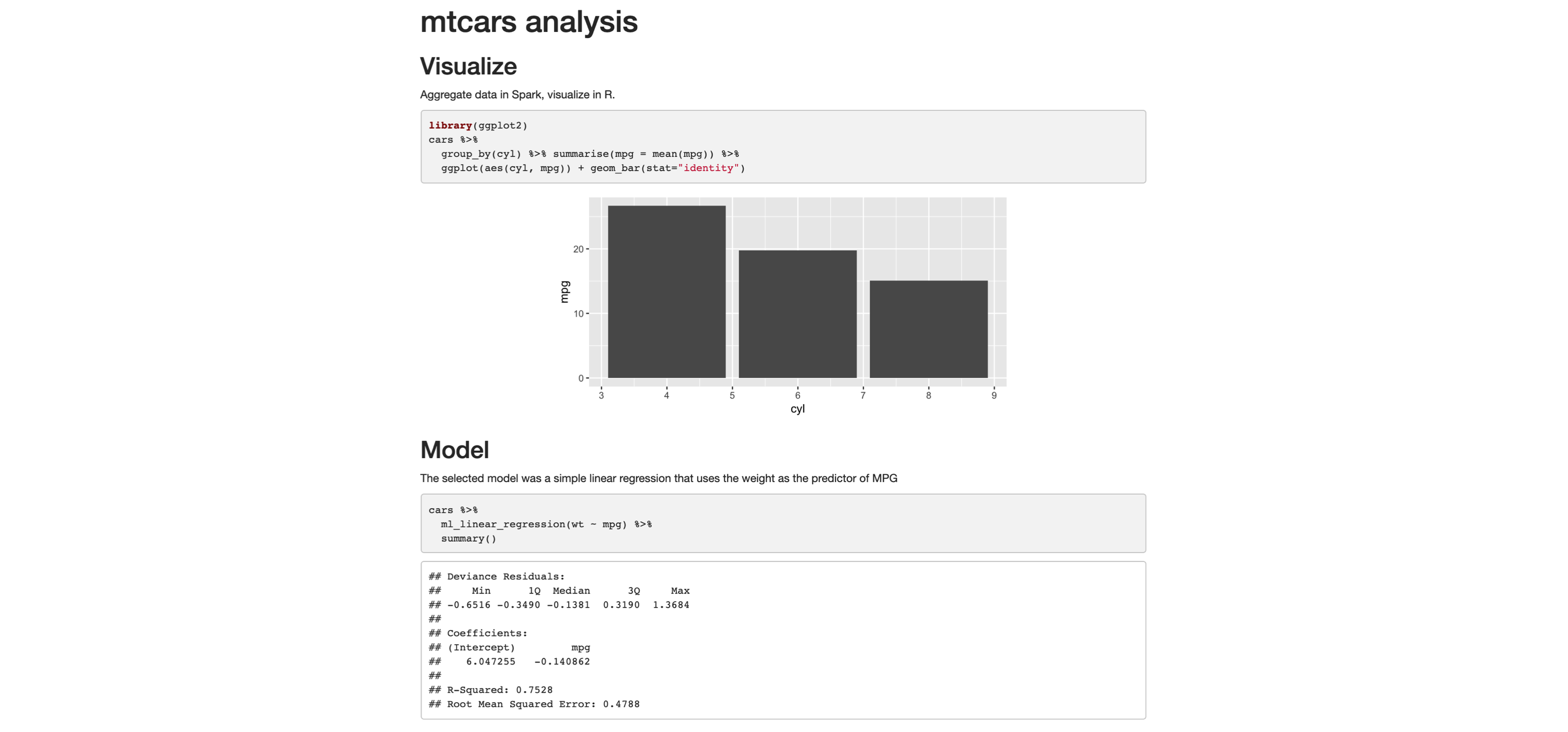R Markdown HTML output||visualize-analysis-communicate

This report can now be easily shared, viewers of this report won’t need Spark and neither R to read and consume its contents, it’s just a self-contained HTML file trivial to open in any browser.

It is also common to distill insights of a report into many other output formats. Switching is quite easy, in the top Front Matter, change the output option to powerpoint_presentation, pdf_document, word_document, etc. Or you can even produce multiple output formats from the same report:

---
title: "mtcars analysis"
output:
word_document: default
pdf_document: default
powerpoint_presentation: default
---

The result will be a PowerPoint presentation, a Word document and a PRD! All of the same information that was displayed in the original HTML report, computed in Spark and rendered in R.

There will be a need to edit the PowerPoint template or the output of the code chunks. This minimal example shows how easy it is to go from one format to another. Of course, it will take some more editing on the R user side to make sure the slides contain only the pertinent information. The main point is to highlight that it does not require to learn a different markup, or code conventions, to switch from one artifact to another.

## 3.7 Recap

This chapter presented a solid introduction to data analysis with R and Spark; many of the techniques presented looked quite similar to using just R and no Spark; which while anticlimactic, it’s the right design to help users already familiar with R to easily transition to Spark. For users unfamiliar with R, this chapter also served as a very brief introduction to some of the most popular (and useful!) packages available in R.

It should now be quite obvious that together, R and Spark, are a powerful combination – a large-scale computing platform, along with an incredibly robust ecosystem of R packages, makes up for an ideal analysis platform.

While doing analysis in Spark with R, remember to push computation to Spark, focus on collecting results in R. Which you can then use for further data manipulation, visualization and communication by sharing your results in a variety of outputs.

The next chapter, Modeling, will dive deeper into how to build statistical models in Spark using a much more interesting dataset, what’s more interesting than dating datasets? You will also learn many more techniques we did not even mention in the brief modeling section from this chapter.

### References

Wickham, Hadley, and Garrett Grolemund. 2016. R for Data Science: Import, Tidy, Transform, Visualize, and Model Data. O’Reilly Media, Inc.

Chang, Winston. 2012. R Graphics Cookbook: Practical Recipes for Visualizing Data. O’Reilly Media, Inc.

Xie, Grolemund, Allaire. 2018. R Markdown: The Definite Guide. 1st ed. CRC Press.